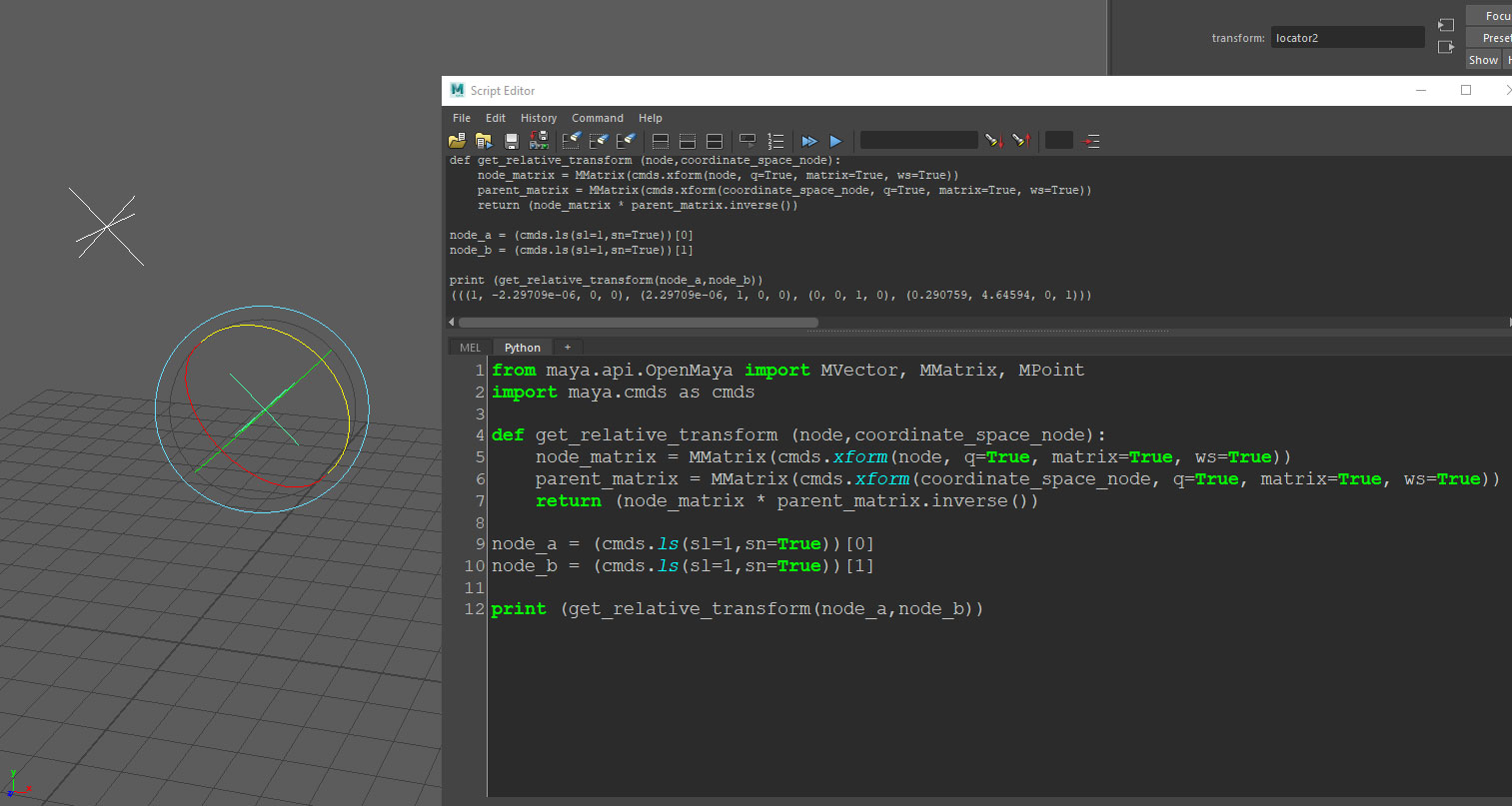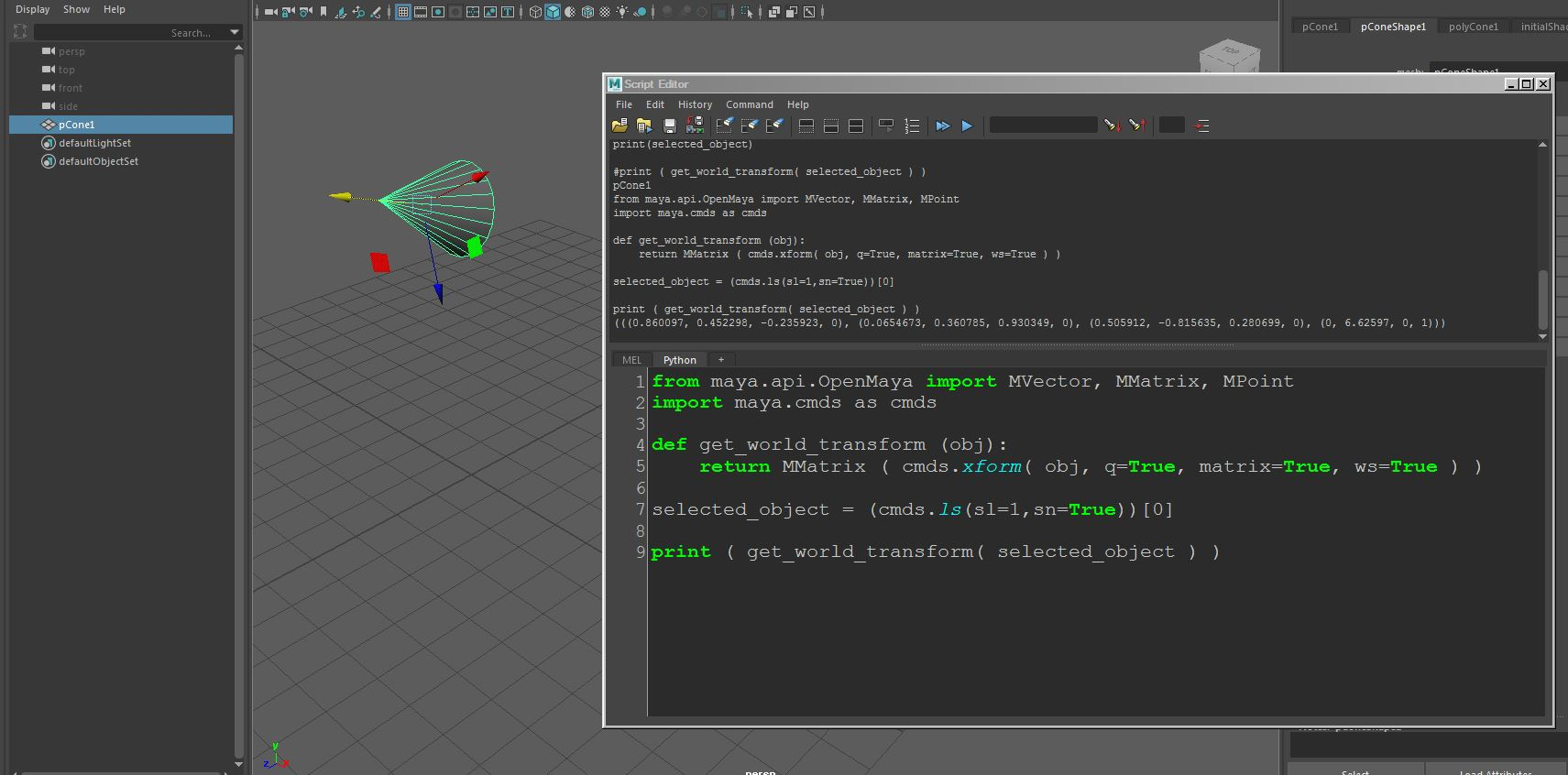## Python – Some useful list-of-tuples operations

Language:
Python 3.7

Sometimes we need to sort a list of tuples (or other list-type containers) according to their internal values.
For instance, you might have a list of tuples representing X,Y coordinates and need to sort that list according to the Y coordinate of the locations.
Using the sorted function with a lambda function as the supplied key argument can do that.
In this case, each tuple element is fed to the lambda function as the argument x and the function simply returns x, which is the second (Y) value within the tuple as the key for the sorting comparison:

`sorted(my_tuple_list, key=lambda x: x)`

Again, in a list of tuples (or other list-type containers), Sometimes we need to find the index of the first occurrence of a tuple that has a specific value as one of its elements.
This example will return the index of the first occurrence in the list of a tuple with the value 8 as its second element:

`list(zip(*my_tuple_list)).index(8)`

The zip function “decouples” the tuples into two lists one containing the tuples first element values and the second containing the tuples second element values.
The zip object is fed into a list function to be converted into a subscript-able list containing the 2 new lists, so now we can use the  index to access the list containing only the original tuples’s second elements, and use the index function to get the index of first occurrence of value 8.
> Note that the * operator isn’t a C like “pointer” operator..
Its a Python unpack operator needed to unpack the list of tuples to separate tuple arguments for the zip function.

## Maya – Basic UV unwrapping

Software:
Maya 2018

Steps for basic UV unwrapping:

1. Select all polygons.
2. Apply planar UV projection just to get rid of all the current UV seams.
3. Select the edges that that are intended to be UV seams.
4. In the UV Editor menu choose Cut/Sew > Cut.
5. Select all polygons.
6. In the UV Editor menu choose Modify > Unfold.
7. In the UV Editor menu choose Modify > Layout.
8. Scale and rotate the UV layout to fit the UV space.

Related:
Multiple UV layouts

## Maya Python scripting – Getting an object’s transform matrix relative to another object’s coordinates

Software:
Maya 2018

How to get an object transformation matrix relative to another object’s coordinates:
* The following script requires selecting 2 objects, the function will return the transform matrix of the first object relative to the transform matrix of the second.

```from maya.api.OpenMaya import MVector, MMatrix, MPoint
import maya.cmds as cmds

def get_relative_transform (node,coordinate_space_node):
node_matrix = MMatrix(cmds.xform(node, q=True, matrix=True, ws=True))
parent_matrix = MMatrix(cmds.xform(coordinate_space_node, q=True, matrix=True, ws=True))
return (node_matrix * parent_matrix.inverse())

node_a = (cmds.ls(sl=1,sn=True))
node_b = (cmds.ls(sl=1,sn=True))

print (get_relative_transform(node_a,node_b))```## Maya Python scripting – Getting an Object’s world-space transform matrix

Software:
Maya 2018

How to get an object transformation matrix in world space coordinates:

```from maya.api.OpenMaya import MVector, MMatrix, MPoint
import maya.cmds as cmds

def get_world_transform (obj):
return MMatrix ( cmds.xform( obj, q=True, matrix=True, ws=True ) )

selected_object = (cmds.ls(sl=1,sn=True))

print ( get_world_transform( selected_object ) )```